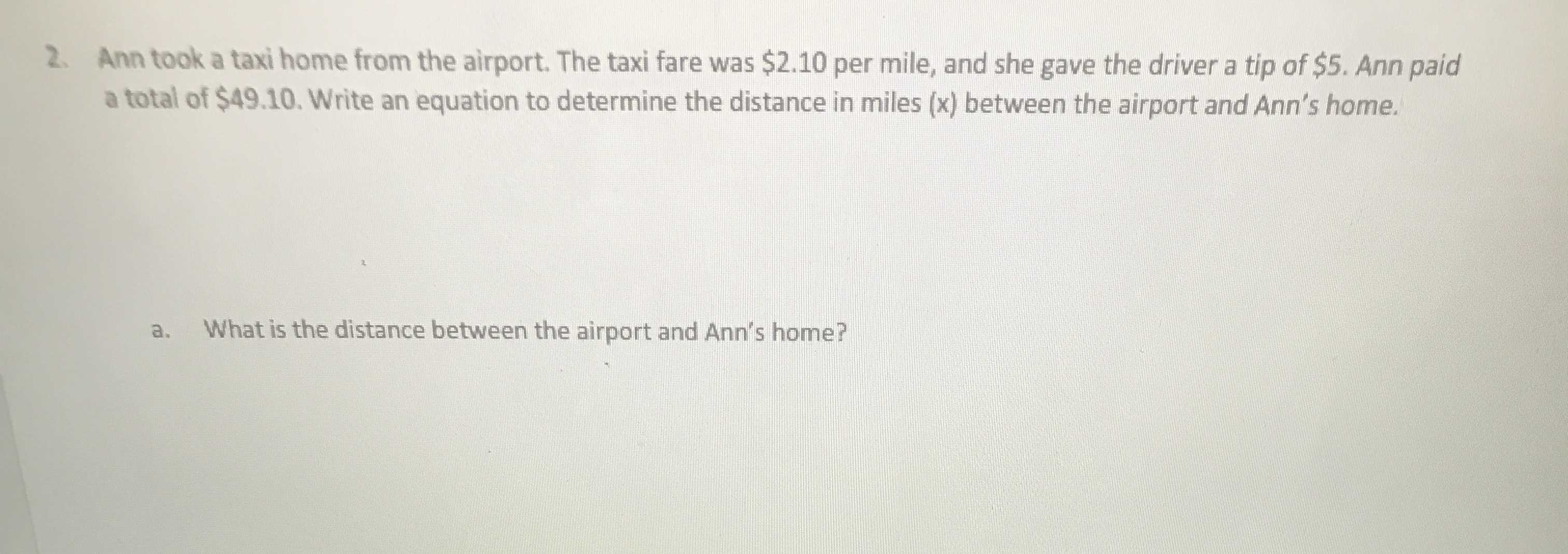### ¿Todavía tienes preguntas de matemáticas?

Pregunte a nuestros tutores expertos
Algebra
Pregunta2. Ann took a taxi home from the airport. The taxi fare was $$\ 2.10$$ per mile, and she gave the driver a tip of $$\ 5$$ . Ann paid a total of $$\ 49.10$$ . Write an equation to determine the distance in miles ( $$x$$ ) between the airport and Ann's home. a. What is the distance between the airport and Ann's home?

x=$$\frac{49.1- 5}{2.1}$$